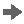Show TOC

###Target / Actual ComparisonReport with which you can analyze the target and actual data in the following application components of Product Cost Controlling using product drilldown:

• Product Cost by Period

• Product Cost by Order

The reporting time frame can be cumulative or restricted.

This report compares the target and actual data that was determined during variance calculation. The results of variance calculation are based on the target cost versions specified for the report: Different variances are considered, depending on the target cost version.

#### Prerequisites

Make sure that the variances have been calculated for the specific target cost version in the relevant reporting time frame.

Reports of summarized analysis require that a data collection run be executed beforehand.

#### Features

##### Data Displayed

The following data is shown based on key figures and/or original cost elements:

• Target Costs

• Actual Costs

• Target Costs Per Unit

• Actual Costs Per Unit

• Absolute Variances = Actual Costs – Target Costs

• Percentage Variances = Absolute Variances ÷ Target Costs

The variances can also be displayed per unit.

The above figures are based on the costs per unit of output.

• Target Quantity

• Confirmed Quantity

• Absolute Variances = Target Quantity – Confirmed Quantity

• Percentage Variances = Absolute Variances ÷ Confirmed QuantityRecommendation